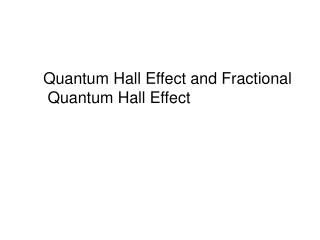DownloadDownload PresentationQuantum Hall Effect and Fractional Quantum Hall Effect

# Quantum Hall Effect and Fractional Quantum Hall Effect

Download Presentation## Quantum Hall Effect and Fractional Quantum Hall Effect

- - - - - - - - - - - - - - - - - - - - - - - - - - - E N D - - - - - - - - - - - - - - - - - - - - - - - - - - -
##### Presentation Transcript

1. Quantum Hall Effect and Fractional Quantum Hall Effect

2. the Lorentz force Hall effect and magnetoresistance Edwin Herbert Hall (1879): discovery of the Hall effect the Hall effect is the electric field developed across two faces of a conductor in the direction j×H when a current j flows across a magnetic field H in equilibrium jy= 0 → the transverse field (the Hall field) Ey due to the accumulated charges balances the Lorentz force quantities of interest: magnetoresistance (transverse magnetoresistance) Hall (off-diagonal) resistance resistivity Hall resistivity the Hall coefficient RH→ measurement of the sign of the carrier charge RHis positive for positive charges and negative for negative charges

3. multiply by the Drude model DC conductivity at H=0 RH→ measurement of the density force acting on electron equation of motion for the momentum per electron in the steady state px and py satisfy cyclotron frequency frequency of revolution of a free electron in the magnetic field H at H = 0.1 T the resistance does not depend on H weak magnetic fields – electrons can complete only a small part of revolution between collisions strong magnetic fields – electrons can complete many revolutions between collisions j is at a small angle fto E f is the Hall angle tan f = wct

4. Higher Mobility= fewer localized states

5. Single electron in the lowest Landau level Filled lowest Landau level

6. Modulation doping and high mobility heterostructures

7. This was just the beginning of high mobilities

8. At high magnetic fields, electron orbits smaller than electron separation

9. new quantum Hall state found at fractional filling factor 1/3

10. Even higher mobilities result in even more fractional quantum Hall states

11. Uncorrelated ? = 1/3 state Correlated ? = 1/3 state Whole new concept of a “Composite Fermion”